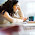## Wednesday, April 10, 2013

### Predicting evaluability: An example application of Decision Tree models

The project: In 2000 ITAD did an Evaluablity Assessment of Sida funded democracy and human rights projects in Latin America and South Africa. The results are available here:Vol.1 and Vol.2. Its a thorough and detailed report.

The data: Of interest to me were two tables of data, showing how each of the 28 projects were rated on 13 different evaluablity assessment criteria. The use of each of these criteria are explained in detail in the project specific assessments in the second volume of the report.

Here are the two tables. The rows list the evaluability criteria and the columns list the projects that were assessed. The cell values show the scores on each criteria: 1 = best possible, 4 = worst possible. The bottom row summarises the scores for each project, and assumes an equal weighting for each criteria, except for the top three, which were not included in the summary score.

CLICK ON THE TABLE TO VIEW AT FULL SIZE

The question of interest: Is it possible to find a small sub-set of these 13 criteria which could act as good predictors of likely evaluability? If so, this could provide a quicker means of assessing where evaluablity issues need attention.

The problem: With 13 different criteria there are conceivably 2 to the power of 13 possible combinations of criteria that might be good predictors i.e 8,192 possiblities

The response:  I amalgamated both tables into one, in an Excel file, and re-calculated the total scores, by including scores for the first three criteria (recoded as Y=1, N=2). I then recoded the aggregate score into a binary outcome measure, where 1 = above average evaluablity scores and 2 below average scores.

I then imported this data into Rapid Miner, an open source data mining package. I then used the Decision Tree module within that package to generate the following Decision Tree model, which I will explain below.

CLICK ON THE DIAGRAM TO VIEW AT FULL SIZE

The results: Decision Tree models are read from the root (at the top) to the leaf, following each branch in turn.

This model tells us, in respect to the 28 projects examined, that IF a project scores less than 2.5 (which is good) on "Identifiable outputs"  AND if it scores less than 3.5 on "project benefits can be attributed to the project intervention alone"  THEN there is a 93% probability that the project is reasonably evaluable (i.e has above average aggregate score for evaluability in the original data set). It also tells us that 50% of all the cases (projects) meet these two criteria.

Looking down the right side of the tree we see that IF the project scores more than 2.5 (which is not good) on"Identifiable outputs" AND even though it scores less than 2.5 on "broad ownership of project purpose amongst stakeholders THEN there is a 100% probability that the project will have low evaluability. It also tells us that 32% of all cases meet these two criteria.

Improvements: This model could be improved in two ways. Firstly, the outcome measure, which is an above/below average aggregate score for each project could be made more demanding, so that only the top 25th percentile were rated as having good evaluability. We may want to set a higher standard.

Secondly, the assumption that all criteria are of equal importance, and thus their scores can simply be added up, could be questioned. Different weights could be given to each criterion, according to their perceived causal importance (i.e. the effects they will have). This will not necessarily bias the Decision Tree model towards using those criteria in a predictive model. If all projects were rated highly on a highly weighted criteria that criteria would have no particular value as a means of discriminating between them, so it would be unlikely to feature in the Decision Tree at all.

Weighting and perhaps subsequent re-weighting criteria may also help reconcile any conflict between what are accurate prediction rules and what seems to make sense as a combination of criteria that will cause high or low evaluability. For example in the above model, it seems odd that a criteria of merit (broad ownership of project purpose) should help us identify projects that have poor evaluablity.

PS: For a pop science account of predictive modelling see Eric Siegel's book on Predictive Analytics

1.2.Nice post . Keep updating Artificial intelligence Online Trining

3.Decision trees are a great flow chart tree structuecire.Yet decision trees are less appropriate for estimation tasks where the goal is to predict the value of a continuous attribute.
To understand further more lets look at some Decision Tree Examples in the Creately diagram community.

4.There is more than one type of "Decision Tree". Yours is a different type to that used for prediction modelling - which is my interest. With Rapid Miner Studio you develop prediction models using both continuous and dichtomous attributes. But thanks for the link to Creately